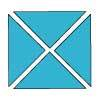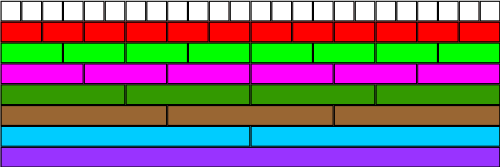#### You may also like### Chocolate

There are three tables in a room with blocks of chocolate on each. Where would be the best place for each child in the class to sit if they came in one at a time?### Four Triangles Puzzle

Cut four triangles from a square as shown in the picture. How many different shapes can you make by fitting the four triangles back together?### Cut it Out

Can you dissect an equilateral triangle into 6 smaller ones? What number of smaller equilateral triangles is it NOT possible to dissect a larger equilateral triangle into?

# Fractional Wall

## Fractional WallUsing the image above, how many different ways can you find of writing $\frac{1}{2}$?

From the picture, what equivalent fractions for $\frac{1}{3}$ can you find?

Again, using the image of the fraction wall, how else could you write $\frac{3}{4}$?

What other fractions do you know that are the same as $\frac{1}{2}$?

Find some other fractions which are equivalent to $\frac{3}{4}$.

Can you find any "rules" for working out equivalent fractions?

You might find it helpful to print off a picture of the fraction wall.

### Why do this problem?

This problem could be used as an introduction to equivalent fractions. It provides a powerful visual representation, helping children develop an understanding of the relationship between different fractions. It gives pupils the opportunity to explore fractions in a non-threatening, open-ended way, encouraging them to develop their own 'rules' for finding equivalent fractions.

### Possible approach

Introduce the activity by showing the fraction wall to the class. Ask the children what they can see and invite them to talk about it in pairs, then share their ideas with the rest of the group. You could steer the conversation towards fractions if the children do not naturally bring it up. Ask general questions about the fractions represented by the different bars in the design, taking the purple bar as the 'whole'. Can pupils spot any fractions which are equivalent? Prompts such as "How many quarters is the same as a half?" might encourage children to think about putting the bars together to make fractions such as $\frac{2}{4}$, rather than just thinking about the unit fractions represented by individual bars.

Allow some time for pupils to work in pairs to find equivalent fractions using the fraction wall. A printable version is available here: Wordpdf. Encourage them to write these fractions down and to see what they notice about the numerator and denominator of the equivalent fractions. As a plenary, ask children to share what they have noticed. Has anybody found a 'rule' for finding equivalent fractions without a picture? Why does this rule work?

### Key questions

What fraction of the purple bar does each blue bar represent? How do you know?
How many sixths is the same as a third?
Write down the equivalent fractions that you have found. What do you notice?

### Possible extension

Once children have completed this activity, they might like to have a go at Fractions Made Faster.

### Possible support

Some learners may find it helpful to draw lines on the fraction wall or cut out the different fractions to check that they are identical. Using Cuisenaire rods to represent the fractions (perhaps choosing a rod of length 6 or 8 to represent the whole) would provide a more concrete fraction environment for children to explore.